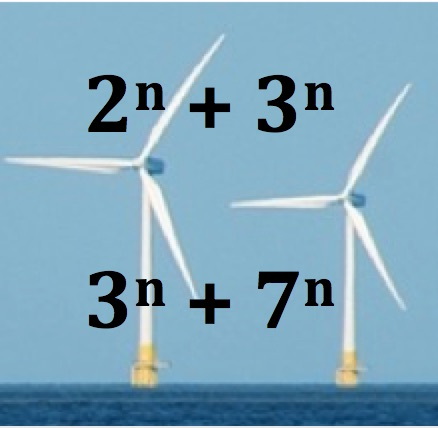Investigate the last digits of the numbers in the picture when n = 1, 2, 3 , 4 and 5. What do you notice? Does this pattern continue? Why?

Will the first number $2^n + 3^n$ be a power of 10 for any value of n?

What can you say about the values of n that make the second number $3^n + 7^n$ a power of 10?

Are there any other pairs of integers between 1 and 10 which have similar properties?